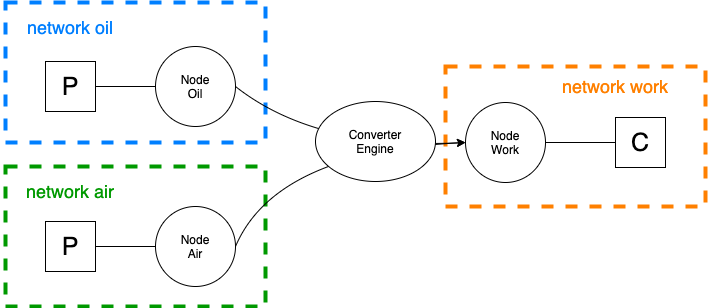# Multi-Energies¶

Hadar is designed to manage many kind of energy. Indeed, the only restriction is the mathematical equation applies on each node, if user case fill in this equation, Hadar can handle user case.

That means Hadar is not designed for a specific energy. And moreover, Hadar can handle many energies in one study, called multi-energies. To do that, Hadar use network which organize node inside the same energy. Node inside the same network manage the same energy, we use Link to plug them together. If user has many network, therefore many energies, he has to use Converter. Converter is more powerfull than Link, user can specify conversion from many different nodes to one node.

## Engine example¶

#### Set problem data

No electricity in this tutorial, we will modelize an explosion engine. There are three kind of energies in an engine: oil (gramme), compressed air (gramme) and work (Joule).figure

Data problem: - 1g of oil = 41868J - for engine, ratio oil/air is 15:1, 1g of oil for 15g of air - engine has an efficiency about 36%

### Find Hadar ratios¶

In Hadar, we have to set ratio $$R_i$$ such as $$In_i * R_i = Out$$ for each input $$i$$.

Equation applies to oil conversion gives:

$\begin{split}\begin{array}{rrcl} &In_{oil} * R_{oil} &=& Work \\ With\ 1g\ of\ oil,& 1 * R_{oil} &=& 41868 * 0,36 \\ &R_{oil} &=& 15072.5 \\ \end{array}\end{split}$

Equation applies to air conversion gives:

$\begin{split}\begin{array}{rrcl} &In_{air} * R_{air} &=& Work \\ Replace\ with\ first\ equation,&In_{air} * R_{air} &=& In_{oil} * R_{oil} \\ With\ 1g\ of\ oil,& 15 * R_{air} &=& 1 * R_{oil} \\ &R_{air} &=& R_{oil} / 15 \\ &R_{air} &=& 1005 \end{array}\end{split}$
import hadar as hd
import numpy as np


Work is modellized by a consumption such as $$10000*(1-e^{-t/25})$$

work = 10000*(1 - np.exp(-np.arange(100)/25))

study = hd.Study(horizon=100)\
.network('work')\
.node('work')\
.consumption(name='work', cost=10**6, quantity=work)\
.network('oil')\
.node('oil')\
.production(name='oil', cost=10, quantity=10)\
.to_converter(name='engine', ratio=15072.5)\
.network('air')\
.node('air')\
.production(name='air', cost=10, quantity=150)\
.to_converter(name='engine', ratio=1005)\
.converter(name='engine', to_network='work', to_node='work', max=10000)\
.build()

optim = hd.LPOptimizer()
res = optim.solve(study)

agg = hd.ResultAnalyzer(study=study, result=res)
plot = hd.HTMLPlotting(agg=agg, unit_symbol='J')

plot.network('work').node('work').stack()